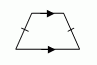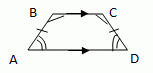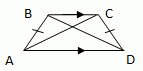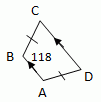Name: ___________________Date:___________________

 Email us to get an instant 20% discount on highly effective K-12 Math & English kwizNET Programs!

### Geometry3.12 Trapezoid

 Trapezoid Is a quadrilateral with exactly one pair of parallel sides. The parallel sides are the bases A trapezoid has two pairs of base angles. For example in the trapezoid ABCD,ĐA and ĐB are one pair of base angles, and ĐC and ĐD are the second pair. The non parallel sides are the legs of the trapezoid. Isosceles Trapezoid: If the legs of the trapezoid are congruent then the trapezoid is an Isosceles trapezoid.THEOREMS: If a trapezoid is isosceles, then each pair of base angles is congruent. If trapezoid ABCD is isosceles, then ĐA @ ĐD and ĐB @ ĐC.If a trapezoid has a pair of congruent base angles, then it is an isosceles trapezoid ABCD is isosceles. If ĐA @ ĐD and ĐB @ ĐC. then ABCD is isosceles.A trapezoid is isosceles if and only if its diagonals are congruent. Trapezoid ABCD is isosceles if and only if AC @ BD.Midsegment Theorem for Trapezoids: The midsegment of a trapezoid is parallel to each base and its length is one half the sum of the lengths of the bases. If MN is the midsegment of trapezoid ABCD, then MN // AB, MN // DC, and MN = 1/2(AB+CD)Directions: 1) For the trapezoid ABCD shown below find the measures of angle A, angle C and angle D.2) Answer the following questions and write at least 5 examples of your own.
 Q 1: A trapezoid is a quadrilateral with exactly ___ pair of parallel sides.onethreetwo Q 2: The parallel sides of a trapezoid are called the ______.legsbasessideslengths Q 3: A trapezoid has two pairs of _____ angles.baseleg Q 4: If the legs of a trapezoid are congruent, then the trapezoid is an ________________.isosceles trapezoidscalene trapezoidequilateral trapezoid Question 5: This question is available to subscribers only! Question 6: This question is available to subscribers only!